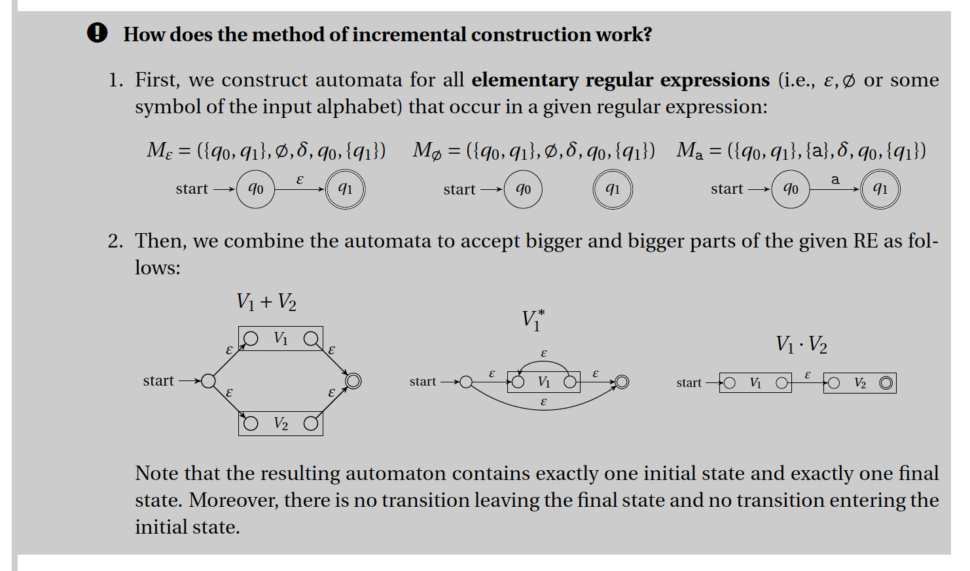Errata

AUTOMATA AND GRAMMARS: A COLLECTION OF EXERCISES AND SOLUTIONS

First Edition, First Reprint (2020)

All errors listed below in the First edition from 2018 have been corrected in this version.

First Edition (2018)

• Page 16:
Exercise 2.2, terminal symbol "r" appears twice in the set of terminal symbols.
• Page 17:
Top grey frame that describes how to create a regular grammar for the concatenation of languages: The algorithm does not work for cases, where grammar G2 includes the following rule: S2 -> epsilon.

Exercise 2.3, terminal symbol "r" appears twice in the set of terminal symbols.
• Page 75:
Starting at the winter semester 2020/2021, we teach the method of incremental construction a little differently. See the new algorithm below.• Page 119:
Exercise 2.156, Step 2(c): the implication symbol should be followed by a^i b^i is not equivalent with a^j b^i.
• Page 132:
Exercise 3.17, there is missing the terminal symbol "c" in grammar G.
• Page 133:
Exercise 3.20 -- the grammar is not correct. For example, it cannot generate bbcb. Correct production rules:
S -> bS | Sa | Sb | ASB | cB
A -> bA | Ab | a
B -> aB | Ba | bB | Bb | b
• Page 136:
Exercise 3.26, the grammar is incorrect. It is not possible to generate part w containing only symbols b (and no symbol a). For example, we cannot generate the string bcb. We can fix this by adding the following rule: W->epsilon.
• Page 144:
In the definition of a left-recursive symbol, there should be used "=>+" instead of "=>*".
• Page 144:
Second grey frame, Step 1. To transform context free grammar to be proper with no simple rules, we should do the following: first, exclude epsilon-rules, second, exclude simple rules, third, exclude redundant symbols. Different order may not lead to proper context-free grammar with no simple rules.
• Page 147:
Grey frame, Step 1. To transform context free grammar to be proper with no simple rules, we should do the following: first, exclude epsilon-rules, second, exclude simple rules, third, exclude redundant symbols. Different order may not lead to proper context-free grammar with no simple rules.
• Page 190 + 191
First grey frame, Page 190: "Turing machine halts if and only if it goes to a final state. Turing machine never halts in a non-final state." This is true if and only if the given Turing machine has total transition function. If the transition function is partial, then the Turing machine can also halt in a non-final state (in case there is no possible transition it can use).
Last grey frame, Page 191: "For every string w not in L: The Turing machine ends up in a final state (i.e., it halts), but the input tape is not empty (there is not a blank symbol B in every cell)." This is again true only if the Turing machine has total transition function. If it is partial, then the Turing machine can also reject strings by halting in a non-final state (in case there is no possible transition it can use).
• Page 196:
Polynomial-time reduction: "Basically, we show that if we know the answer for the problem A, then we are able (in polynomial-time) to find the answer for the problem B." - This sentence may be confusing. Its meaning is the following: If we have some instance of problem A and if we know whether it is yes or no-instance, then we can convert this instance in polynomial time into an instance of problem B with the same truth value (if the initial instance of problem A was yes-instance, then the resulting instance of problem B will also be yes-instance, similarly for no-instance).

The following is also true for polynomial-time reduction: If we know some polynomial-reduction of a problem A to a problem B, then A is at most as hard as B. We always reduce to a problem that is at least as hard. Therefore, if we can reduce A to B in polynomial-time and if we can solve B in polynomial-time, then we can solve A in polynomial-time (since A is at most as hard as B).

Didn't find what you were looking for? Did you find some parts difficult to understand? Did you miss something in the textebook or do you have any ideas how to improve it?

Vaše osobní údaje budou použity pouze pro účely vyřešení vašeho dotazu.